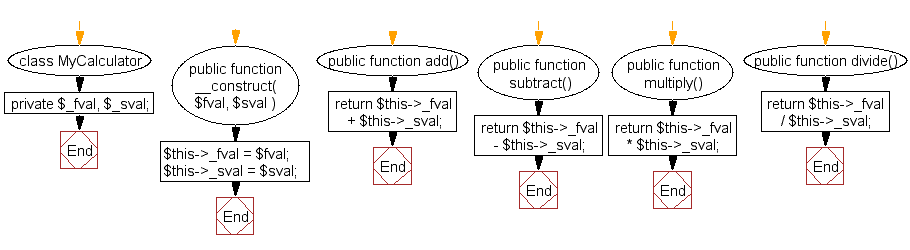﻿ PHP Class Exercise: Calculator class which will accept two values as arguments - w3resource# PHP Class Exercises : Calculator class which will accept two values as arguments

## PHP class: Exercise-6 with Solution

Write a PHP calculator class which will accept two values as arguments, then add them, subtract them, multiply them together, or divide them on request.

For example :
\$mycalc = new MyCalculator( 12, 6);
echo \$mycalc- > add(); // Displays 18
echo \$mycalc- > multiply(); // Displays 72

Sample Solution:

PHP Code:

``````<?php
class MyCalculator {
private \$_fval, \$_sval;
public function __construct( \$fval, \$sval ) {
\$this->_fval = \$fval;
\$this->_sval = \$sval;
}
return \$this->_fval + \$this->_sval;
}
public function subtract() {
return \$this->_fval - \$this->_sval;
}
public function multiply() {
return \$this->_fval * \$this->_sval;
}
public function divide() {
return \$this->_fval / \$this->_sval;
}
}
\$mycalc = new MyCalculator(12, 6);
echo \$mycalc-> add()."\n"; // Displays 18
echo \$mycalc-> multiply()."\n"; // Displays 72
echo \$mycalc-> subtract()."\n"; // Displays 6
echo \$mycalc-> divide()."\n"; // Displays 2
?>
```
```

Sample Output:

```18
72
6
2
```

Flowchart :PHP Code Editor:

Have another way to solve this solution? Contribute your code (and comments) through Disqus.

What is the difficulty level of this exercise?

﻿

## PHP: Tips of the Day

PHP: Anonymous recursive PHP functions

In order for it to work, you need to pass \$factorial as a reference

```\$factorial = function( \$n ) use ( &\$factorial ) {
if( \$n == 1 ) return 1;
return \$factorial( \$n - 1 ) * \$n;
};
print \$factorial( 5 );
```

Ref : https://bit.ly/38dj7jm